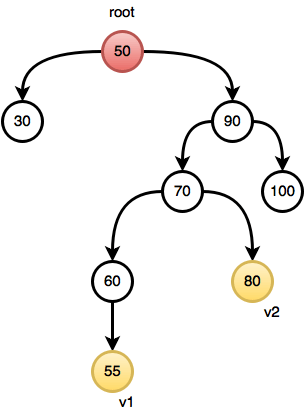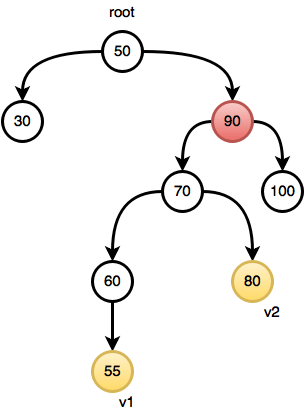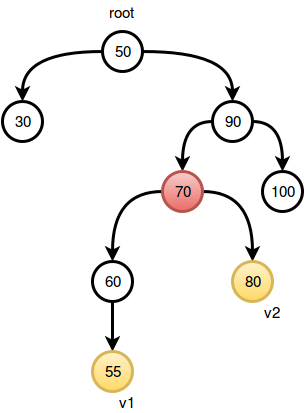# Binary Search Tree : Lowest Common Ancestor Hackerrank

##### Binary Search Tree : Lowest Common Ancestor Hackerrank

You are given pointer to the root of the binary search tree and two values v1 and v2. You need to return the lowest common ancestor (LCA) of v1 and 2 in the binary search tree. You only need to complete the function.

Input Format

You are given a function,

node * LCA (node * root ,int v1,int v2)
{

}

It is guaranteed that v1 and v2 are present in the tree.

Node is defined as :

struct node
{
int data;
node * left;
node * right;
}node;

Output Format

Return the LCA of v1 and v2.

Sample Input

4
/ \
2 7
/ \ /
1 3 6

v1=1 and v2=7.

Sample Output

LCA of 1 and 7 is 4 (which is the root).
Return a pointer to the root in this case.

##### Solution

You have to consider 3 cases to solve this problem.
Case 1: Both v1 and v2 are on the right of the current rootIn this case, you can be sure that the LCA is situated in the right subtree.
Case 2: Both v1 and v2 are on the left of the current rootIn this case, you can be sure that the LCA is situated in the left subtree.
Case 3: v1 and v2 are on two different subtrees or one of them is the current root.In this case, the current root is the LCA, you don’t need to search anymore!
Time complexity is O(depth of the tree).

``````

/* Node is defined as :
class Node
int data;
Node left;
Node right;

*/
static Node lca(Node root,int v1,int v2)
{
//Decide if you have to call rekursively
//Samller than both
if(root.data < v1 && root.data < v2){
return lca(root.right,v1,v2);
}
//Bigger than both
if(root.data > v1 && root.data > v2){
return lca(root.left,v1,v2);
}

return root;
}
``````
``````static Node lca(Node root,int v1,int v2)
{
//Decide if you have to call recursively
//Samller than both
if(root.data < v1 && root.data < v2){
return lca(root.right,v1,v2);
}
//Bigger than both
if(root.data > v1 && root.data > v2){
return lca(root.left,v1,v2);
}